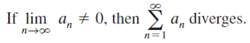# nth Term Test for Divergence

## nth Term Test for Divergence of Series

The nth Term Test for Divergence (also called The Divergence Test) is one way to tell if a series diverges.

• If a series converges, the terms settle down on a finite number as they get larger (towards infinity).
• If a series diverges, then the terms do not get smaller as n gets larger.

The nth term test is formally defined as:In other words, if the limit of the nth term doesn’t equal zero, then the series diverges. To put this another way, ask yourself the question:
Does the sum of the nth term in the series approach zero?
If the answer is no, then the series diverges .

If the limit equals zero, then the nth term test doesn’t apply: you can’t use it to determine convergence or divergence.

There are many different series convergence tests, and the simple nth term test is one of the easiest to try. If you don’t reach a conclusion using this test, then try one of the others.

## Nth Term Test for “Convergence”

The test is sometimes (informally) called the nth Term Test for convergence, but this is misleading because:

• Failing the test guarantees that a series diverges,
• Passing the test does not guarantee that a series converges .

## Nth Term Test Examples

Example #1: Does the infinite series 1 + (−1) + 1 + (−1) + … diverge?
Solution: The limit as n approaches infinity does not exist, so the series diverges.

Example #2: Does the series 2n diverge?
Solution: The limit as n approaches infinity does not equal zero (in fact, the numbers get very large very quickly), so the series diverges.

Example #3: Does the series 1/n diverge?
Solution: The limit as n approaches infinity equals zero, so we cannot conclude anything about whether the series converges or diverges.

## References

 Convergence Tests for Infinite Series. Retrieved April 6, 2021 from: https://www.rit.edu/academicsuccesscenter/sites/rit.edu.academicsuccesscenter/files/documents/math-handouts/C14_ConvergenceTestsForInfiniteSeries_BP_10_8_14.pdf
 Pflueger, N. (2011). Lecture 17: The Nth term test and the comparison test. Retrieved April 6, 2021 from: https://npflueger.people.amherst.edu/math1b/Lecture17.pdf

CITE THIS AS:
Stephanie Glen. "nth Term Test for Divergence" From StatisticsHowTo.com: Elementary Statistics for the rest of us! https://www.statisticshowto.com/nth-term-test-for-divergence/